﻿ Objects > Parametric Plots

# Parametric Plots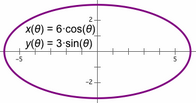A parametric plot graphs two functions on a coordinate system. Each plotted point (x, y) or (r, θ) corresponds to a particular value of an independent variable. This independent variable is used to evaluate each of the two functions. The value of the first function is used for the x or r value, and the value of the second function is used for the y or θ value. (The independent variable is usually called a parameter, although it's not a separate object like a Sketchpad parameter.)

When you define a parametric plot, you choose whether to plot it using rectangular or polar coordinates, and you choose the domain for the independent variable.

You can change the object properties, label properties, and display attributes of a parametric plot.

You can change the line style of a continuous parametric plot, and you can change the point style of a discrete parametric plot.

You can choose Edit | Properties | Plot to change the number of samples used to display a parametric plot and to change whether it’s displayed continuously or discretely.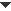Construct a Parametric Plot
 To construct a parametric plot, select two functions and choose Alternatively, you can choose the command and, with the Plot Curve dialog box open, click the functions in the sketch to enter them into the dialog box. The parametric plot appears on the marked coordinate system. If there is no coordinate system, Sketchpad creates one.Use a Parametric Plot
 •Construct a point on a parametric plot using the Point tool or the Construct | Point on Object command. You can then animate the point along the parametric plot or use the point in any other way you like.•Use a parametric plot as a path along which to animate an independent point by using the Edit | Merge command.•Use to construct the intersection of a parametric plot with a straight object, a circle, or an arc.Alternatively, click the intersection with the Arrow tool or with any tool that can construct a point.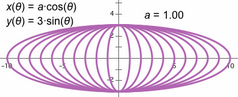•If one or both defining functions depend on a parameter or slider, you can  construct a family of parametric plots. Select both the parametric plot and the parameter or slider and chooseChange the Domain of a Parametric Plot

When you construct a parametric plot, enter the upper and lower limits of the domain in the Plot Curve dialog box.

To change the domain, you can:

Select the parametric plot, choose and enter new values for the limits of the domain.

Use the Arrow tool to drag the arrowheads that appears at the ends of the parametric plot.

Drag in the direction that the arrowhead points to increase the length of the plot; drag in the opposite direction to decrease the length of the plot.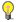Note

 To determine whether the arrowheads appear, chooseChange the Number of Samples
 Mathematically, a parametric plot may include an infinite number of points. However, to display an infinite number of points would require a computer to use an infinite amount of time, so Sketchpad instead plots a large (but not infinite) number points. Each point that Sketchpad does display is called a sample. To change the number of samples for a selected parametric plot: •Press the + or – key. Each press of the key increases or decreases the number of samples.•Choose Increase Resolution or Decrease Resolution from the Context menu. •Choose Edit | Properties | Plot and type a new value for the number of samples.You can change the initial number of samples for newly constructed parametric plot. Choose Edit | Advanced Preferences | Sampling and type a new value for Number of samples for new function plots. (This setting applies to new function plots and parametric plots, but has no effect on already-existing plots.)Display a Parametric Plot Continuously or Discretely

Change between continuous and discrete display of a selected parametric plot by choosing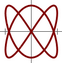Continuous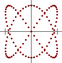Discrete